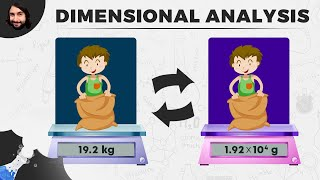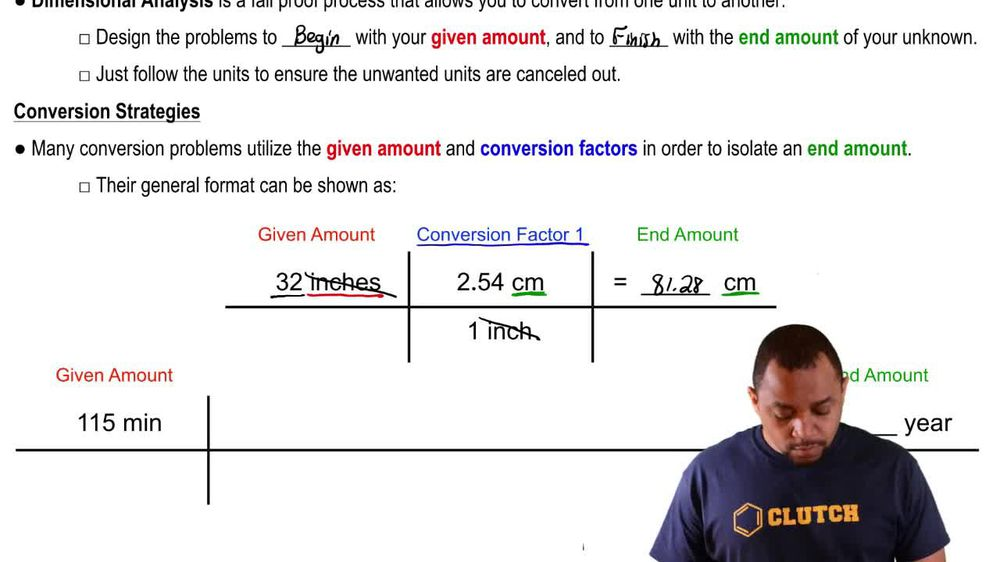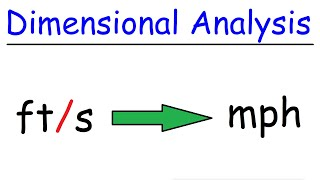Start typing, then use the up and down arrows to select an option from the list.
1. 1. Intro to General Chemistry2. Dimensional Analysis
Problem

# A period at the end of sentence written with a graphite pen-cil has a diameter of 1 mm. How many carbon atoms would it take to line up across the period if a single carbon atom has a diameter of 150 pm?

Relevant Solution1m
Play a video:
Hi everyone today. I have a question asking us to calculate the number of silicon atoms needed to create a 7.0 micro meter line. If the radius of the silicon atom is 210 pick zero m. So we're gonna start off with our 7. micrometers and we're going to change that over to PICO meters. So we're gonna multiply by one. Pick zero m over One times 10 to the negative 6 μ m and then we're going to change that to adam. So we're going to multiply by one atom over 210 PICO m. So our micro meters are going to cancel out. Our PICO meters are going to cancel out and that's going to give us 33333. And if we want to change that to scientific notation, we're going to take our decimal place one 2, 34 to the right, so that equals 3.3 Times 10 to the 4th. And that is our final answer. Thank you for watching. Bye.